SHIVANSH KAUSHAL — Published On July 27, 2022 and Last Modified On August 8th, 2022

## Introduction

Over the past few decades, there has been a tremendous advancement in AI technologies. We can see the heavy usage of these technologies almost everywhere in our lives, from the healthcare industry to self-driving cars to completely automated machines and whatnot. Computer vision is artificial intelligence that enables computers to derive information from images, videos, and other inputs. Before jumping into this cutting-edge technology, let’s discuss the basic building blocks of many edge detection algorithms, Image Gradient.

Let’s understand the term Image Gradient first. Image is a matrix of pixel values representing various intensity level values. A pixel is the building block of an image. The gradient can be defined as the change in the direction of the intensity level of an image.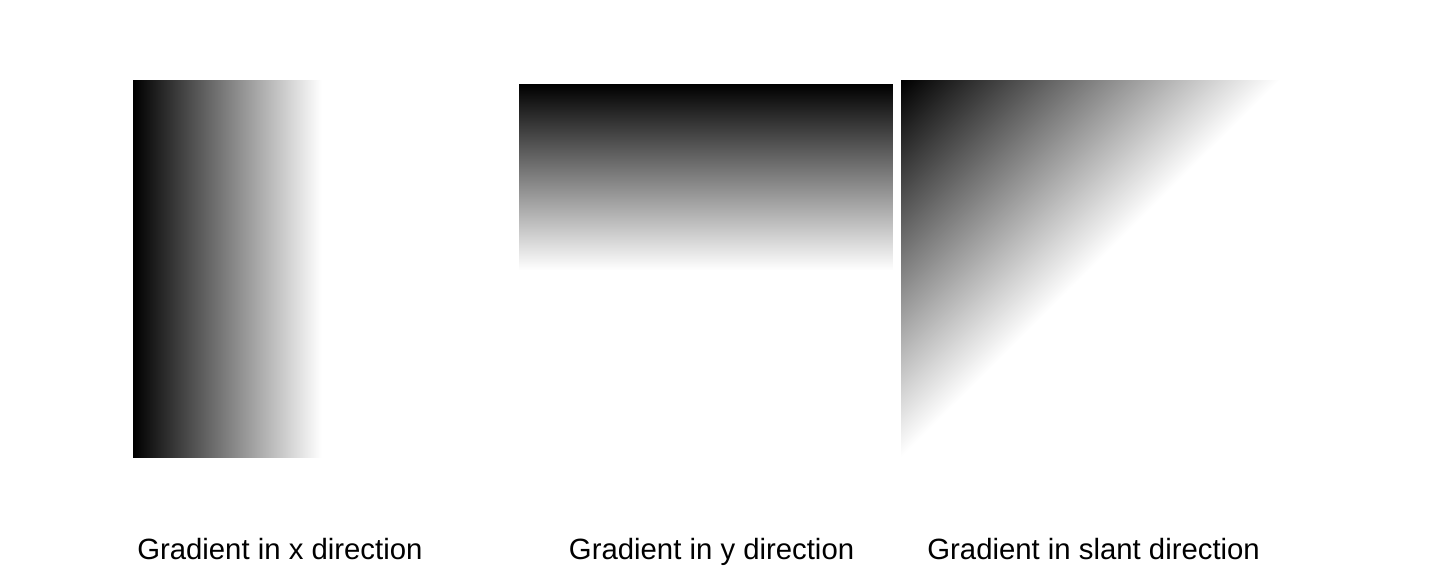So, the gradient helps us measure how the image changes and based on sharp changes in the intensity levels; it detects the presence of an edge. We will dive deep into it by manually computing the gradient in a moment.

## Why do we need an image gradient?

Image gradient is used to extract information from an image. It is one of the fundamental building blocks in image processing and edge detection. The main application of image gradient is in edge detection. Many algorithms, such as Canny Edge Detection, use image gradients for detecting edges.

## Mathematical Calculation of Image gradients

Enough talking about gradients, Let’s now look at how we compute gradients manually. Let’s take a 3*3 image and try to find an edge using an image gradient. We will start by taking a center pixel around which we want to detect the edge. We have 4 main neighbors of the center pixel, which are:

(i) P(x, y-1) top pixel
(ii) P(x+1,y) right pixel
(iii) P(x-1,y) left pixel
(iv) P(x,y+1) bottom pixel

We will subtract the pixels opposite to each other i.e. Pbottom – Ptop and Pright – Pleft , which will give us the change in intensity or the contrast in the level of intensity of the opposite the pixel.

Change of intensity in the X direction is given by:

`                  Gradient in Y direction = PR - PL`

Change of intensity in the Y direction is given by:

`                  Gradient in Y direction = PB - PT`

Gradient for the image function is given by:

`                 𝛥I = [𝛿I/𝛿x, 𝛿I/𝛿y]`

Let us find out the gradient for the given image function:We can see from the image above that there is a change in intensity levels only in the horizontal direction and no change in the y direction. Let’s try to replicate the above image in a 3*3 image, creating it manually-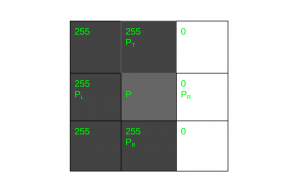Let us now find out the change in intensity level for the image above

```GX = PR - PL Gy = PB - PT
GX = 0-255 = -255
Gy = 255 - 255 = 0
```

Gradient for this Image function will be:
𝛥I = [ -255, 0]

Let us take another image to understand the process clearly.Let us now try to replicate this image using a grid system and create a similar 3 * 3 image.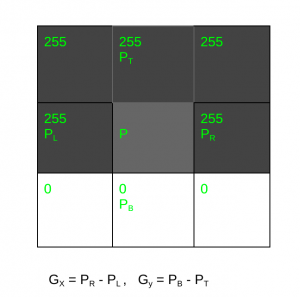Now we can see that there is no change in the horizontal direction of the image

```GX = PR - PL , Gy = PB - PT

GX = 255 - 255 = 0
Gy = 0 - 255 = -255
```

Gradient for this Image function will be:
𝛥I = [0, -255]

But what if there is a change in the intensity level in both the direction of the image. Let us take an example in which the image intensity is changing in both the directionLet us now try replicating this image using a grid system and create a similar 3 * 3 image.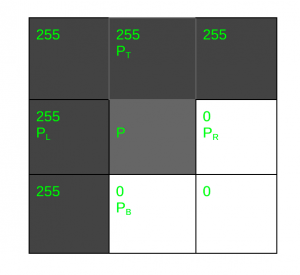```GX = PR - PL , Gy = PB - PT

GX = 0 - 255 = -255
Gy = 0 - 255 = -255
```

Gradient for this Image function will be:
𝛥I = [ -255, -255]

Now that we have found the gradient values, let me introduce you to two new terms:

Gradient magnitude represents the strength of the change in the intensity level of the image. It is calculated by the given formula:

```Gradient Magnitude: √((change in x)² +(change in Y)²)
```

The higher the Gradient magnitude, the stronger the change in the image intensity

Gradient Orientation represents the direction of the change of intensity levels in the image. We can find out gradient orientation by the formula given below:

```Gradient Orientation: tan-¹( (𝛿I/𝛿y) / (𝛿I/𝛿x)) * (180/𝝅)
```

## Overview of Filters

We have learned to calculate gradients manually, but we can’t do that manually each time, especially with large images. We can find out the gradient of any image by convoluting a filter over the image. To find the orientation of the edge, we have to find the gradient in both X and Y directions and then find the resultant of both to get the very edge.

Different filters or kernels can be used for finding gradients, i.e., detecting edges.

3 filters that we will be working on in this article are

• Roberts filter
• Prewitt filter
• Sobel filter

All the filters can be used for different purposes. All these filters are similar to each other but different in some properties. All these filters have horizontal and vertical edge detecting filters.

These filters differ in terms of the values orientation and size

#### Roberts Filter

Roberts filter is a 2 * 2 filter. It is one of the oldest and simplest filters. The idea behind the Roberts cross operator is to approximate the gradient of an image through discrete differentiation, computed by summing the squares of the differences between diagonally adjacent pixels.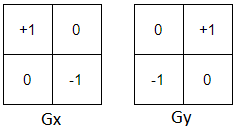Img src: https://miro.medium.com/max/476/1*XkJStqoyMFD60bgeNcjSNg.png

We will now look at how we can find image gradients using filters. We will start with the Roberts filter.
Suppose we have this 4*4 image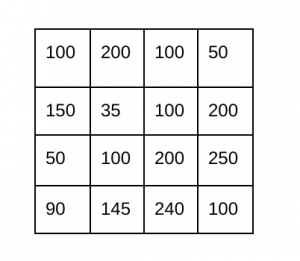Now, will take both the Gx and Gy filters and convolute them over the image.
Let us look at the computation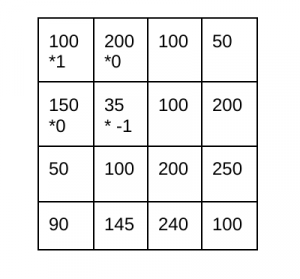```Gx = 100 *1 + 200*0 + 150*0 - 35*1
Gx = 65
```

The gradient in y direction =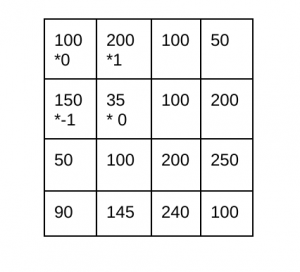```Gy = 100 *0 + 200*1 - 150*1 + 35*0
Gy = 50
```

Now that we have found out both these values, let us calculate gradient strength and gradient orientation.

```Gradient magnitude = √(Gx)² + (Gy)²
= √(65)² + (50)² = √6725 ≅ 82
```

We can use the arctan2 function of NumPy to find the tan-1 in order to find the gradient orientation

```Gradient Orientation = np.arctan2( Gy / Gx) * (180/ 𝝅)
= 37.5685
```

#### Prewitt Filter

Prewitt filter is a 3 * 3 filter and it is more sensitive to vertical and horizontal edges as compared to the Sobel filter. It detects two types of edges – vertical and horizontal. Edges are calculated by using the difference between corresponding pixel intensities of an image.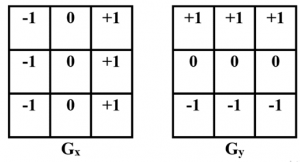#### A working example of Prewitt filter

Let’s calculate the image gradient using the Prewitt filter
Suppose we have the same 4*4 image as earlierNow, I will take both the Gx and Gy filters and convolute them over the image.
Let us look at the computation

The gradient in x direction =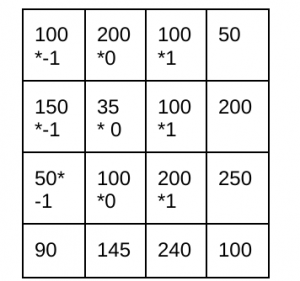```Gx = 100 *(-1) + 200*0 + 100*1 + 150*(-1) + 35*0 + 100*1 + 50*(-1) + 100*0 + 200*1
Gx = 100
```

The gradient in y direction =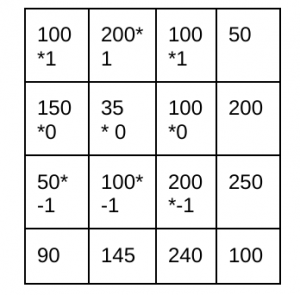```Gy = 100 *1 + 200*1 + 200*1 + 150*0 + 35*0 +100*0 + 50*(-1) + 100*(-1) + 200*(-1)
Gy = 150
```

Now that we have found both these values let us calculate gradient strength and gradient orientation.

```Gradient magnitude = √(Gx)² + (Gy)²
= √(100)² + (150)² = √32500 ≅ 180
```

We will use the arctan2 function of NumPy to find the gradient orientation

```Gradient Orientation = np.arctan2( Gy / Gx) * (180/ 𝝅)
= 56.3099
```

#### Sobel Filter

Sobel filter is the same as the Prewitt filter, and just the center 2 values are changed from 1 to 2 and -1 to -2 in both the filters used for horizontal and vertical edge detection.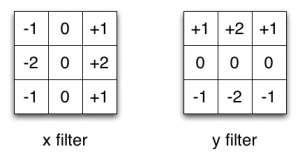Img src: https://blog.e-kursy.it/deeplearning4j-cnn/video/html/

#### A working example of Sobel filter

Let’s calculate the image gradient using the Sobel filter
Suppose we have the same 4*4 image as earlier

Now, I will take both the Gx and Gy filters and convolute them over the image.
Let us look at the computation

The gradient in x direction =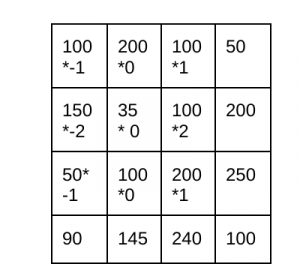```Gx = 100 *(-1) + 200*0 + 100*1 + 150*(-2) + 35*0 + 100*2 + 50*(-1) + 100*0 + 200*1
Gx = 50
```

The gradient in y direction =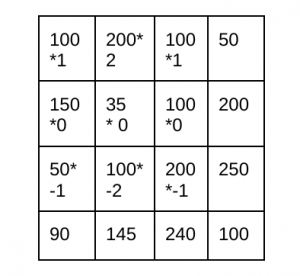```Gy = 100 *1 + 200*2 + 100*1 + 150*0 + 35*0 +100*0 + 50*(-1) + 100*(-2) + 200*(-1)
Gy = 150
```

Now that we have found out both these values, let us calculate gradient strength and gradient orientation.

```Gradient magnitude = √(Gx)² + (Gy)²
= √(50)² + (150)² = √ ≅ 58
```

Using the arctan2 function of NumPy to find the gradient orientation

```Gradient Orientation = np.arctan2( Gy / Gx) * (180/ 𝝅)
= 71.5650
```

## Implementation using OpenCV

Let us now implement the OpenCV code for Image gradient using the abovementioned filters.
We will perform the program on a very famous image known as Lenna.

Let us start by installing the OpenCV package

```#installing opencv
!pip install cv2
```

After we have installed the package, let us import the package and other libraries

Python Code:

#### Using Prewitt Filter

```#Converting image to grayscale
gray_img = cv2.cvtColor(img,cv2.COLOR_BGR2GRAY)

#Creating Prewitt filter
kernelx = np.array([[1,1,1],[0,0,0],[-1,-1,-1]])
kernely = np.array([[-1,0,1],[-1,0,1],[-1,0,1]])

#Applying filter to the image in both x and y direction
img_prewittx = cv2.filter2D(img, -1, kernelx)
img_prewitty = cv2.filter2D(img, -1, kernely)

# Taking root of squared sum(np.hypot) from both the direction and displaying the result
prewitt = np.hypot(img_prewitty,img_prewittx)
prewitt = prewitt[:,:,0]
prewitt = prewitt.astype('int')
plt.imshow(prewitt,cmap='gray')
```

OUTPUT: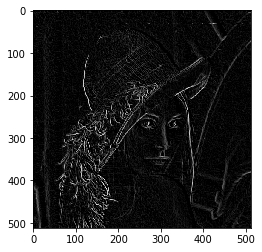#### Using Sobel filter

```gray_img = cv2.cvtColor(img,cv2.COLOR_BGR2GRAY)
kernelx = np.array([[-1,0,1],[-2,0,2],[-1,0,1]])
kernely = np.array([[1, 2, 1],[0, 0, 0],[-1,-2,-1]])
img_x = cv2.filter2D(gray_img, -1, kernelx)
img_y = cv2.filter2D(gray_img, -1, kernely)

#taking root of squared sum and displaying result
new=np.hypot(img_x,img_y)
plt.imshow(new.astype('int'),cmap='gray')
```

OUTPUT: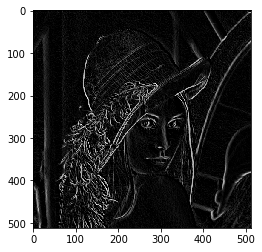## Conclusion

This article taught us the basics of Image gradient and its application in edge detection. Image gradient is one of the fundamental building blocks of image processing. It is the directional change in the intensity of the image. The main application of image gradient is in edge detection. Finding the change in intensity can conclude that it can be a boundary of an object. We can compute the gradient, its magnitude, and the orientation of the gradient manually. We usually use filters, which are of many kinds and for different results and purposes. The filters discussed in this article are the Roberts filter, Prewitt filter, and Sobel filter. We implemented the code in OpenCV, using all these 3 filters for computing gradient and eventually finding the edges.

Some key points to be noted:

• Image gradient is the building block of any edge detection algorithm.
• We can manually find out the image gradient and the strength and orientation of the gradient.
• We learned how to find the gradient and detect edges using different filters coded using OpenCV.